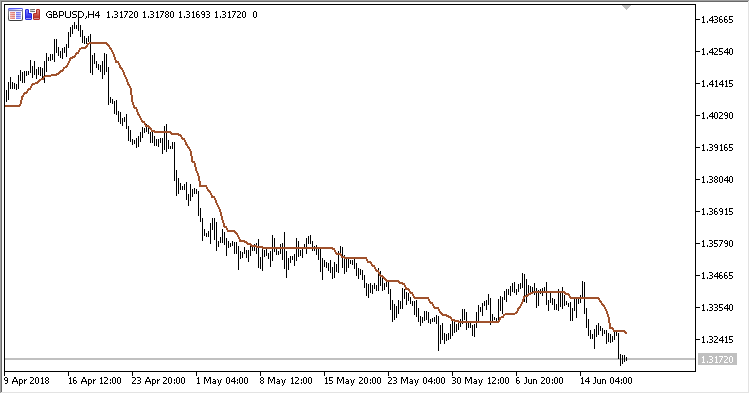# CA – indicator for MetaTrader 5

• A+
category：MT5 INDICATORS

Indicator CA (Corrected Average), also known as Optimal Moving Average. The benefit of the indicator is the fact that the current value of the timeseries must exceed the current threshold that depends on volatility for the indicator line to follow the price, avoiding false signals in the trend.

The indicator has three configurable parameters:

• Period - calculation period;
• Method - calculation method;
• Applied price - price used for calculations.

Calculations:

`CA = PrevCA + k * (MA-PrevCA)`

where:

`MA - MA(Applied price, Period, Method)`

If diff2 > Dev:

`k = 1-Dev / diff2`

otherwise:

`k = 0`
```Dev = StdDev*StdDev
StdDev - Standard deviation(Applied price,Period)
diff2 = diff * diff,
diff = PrevCA - MA
```